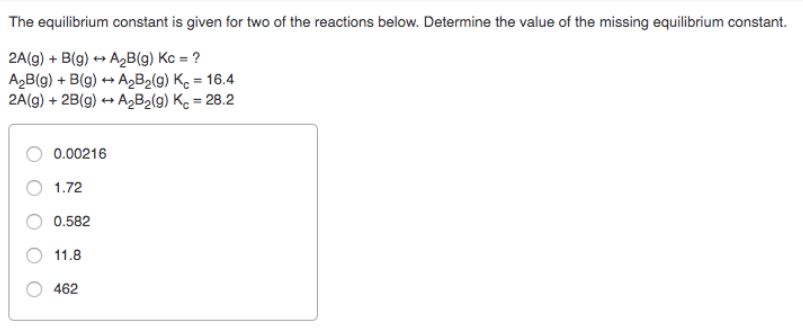# The equilibrium constant is given for two of the reactions below. Determine the value of the missing equilibrium constant. 2A(g) + B(g) <-> A2B(g) Kc = ? A2(g) + B(g) <-> A2B2(g) Kc = 16.4 2A(g) + 2B(g) <-> A2B2(g) Kc = 28.2 a) 0.00216 b) 1.72 c) 0.582 d) 11.8 e) 462